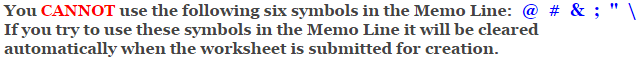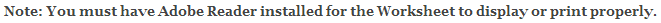Fractions Worksheets

Multiplying Fractions Worksheets

This fraction worksheet is great for great for working on multiplying fractions. The problems may be selected for three different degrees of difficulty.

The answer worksheet will show the progression of multiplying fractions.

This fraction worksheet will generate 10 or 15 multiplying fraction problems per worksheet.

Difficulty of the Multiplying Fractions Problems

Numerators 1 thru 9.
Denominators 2, 3, 4, 5, & 10.

Numerators 1 thru 9.
Denominators 2 thru 10.

Numerators 1 thru 19.
Denominators 2 thru 20.

Language for the Multiplying Fractions Worksheet

 English German Albanian Spanish Swedish Italian French Turkish Polish Norwegian

Memo Line for the Multiplying Fractions Worksheet

You may enter a message or special instruction that will appear on the bottom left corner of the Multiplying Fractions Worksheet.Multiplying Fractions Worksheet Answer Page

Now you are ready to create your Multiplying Fractions Worksheet by pressing the Create Button.

 Recommended Videos

If You Experience Display Problems with Your Math Worksheet